## Mundorf

Mundorf offers a wide range of high quality air motion transformers (AMT).

## AMT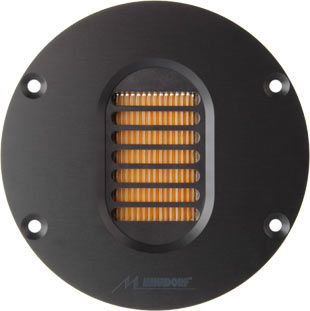open a bigger photo

Mundorf AMT 19CM1.1-C       order no. M-amt19cm11-c       EUR 239,90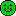excl. VAT: € 201.60 / \$ 227.80
air motion transformer. The magnet system is based on Neodymium technology. The speaker has a 3 mm solid metal front plate.
• power handling (continuous/programme) = 120 W
• frequency range = 1500-38000 Hz
• resonance frequency fs = 2300 Hz
• impedance R = 8 Ohm
• sound pressure level SPL = 91 dB (2,83V; 1m)
• DC resistance Re = 8,1 Ohm
• effective piston radiating area Sd = 126 cm2
• overall diameter d = 104 mm
PDF data sheetopen a bigger photo

Mundorf AMT 19CM2.1-C       order no. M-amt19cm21-c       EUR 179,00excl. VAT: € 150.42 / \$ 169.97
air motion transformer. The magnet system is based on Neodymium technology. The speaker has a 3 mm solid metal front plate.
• power handling (continuous/programme) = 120 W
• frequency range = 1600-38000 Hz
• resonance frequency fs = 2600 Hz
• impedance R = 4 Ohm
• sound pressure level SPL = 93 dB (2,83V; 1m)
• DC resistance Re = 4,2 Ohm
• effective piston radiating area Sd = 126 cm2
• overall diameter d = 104 mm
PDF data sheetMundorf AMT 1908C, D= 94 mm, schwarz       order no. M-amt1908cr94       EUR 209,00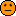excl. VAT: € 175.63 / \$ 198.46
air motion transformer. The magnet system is based on Neodymium technology. The speaker has a 3 mm solid metal front plate.
• power handling (continuous/programme) = 120 W
• frequency range = 1800-41000 Hz
• resonance frequency fs = 2300 Hz
• impedance R = 8 Ohm
• sound pressure level SPL = 91 dB (2,83V; 1m)
• DC resistance Re = 8,1 Ohm
• effective piston radiating area Sd = 126 cm2
• overall diameter d = 94 mm
PDF data sheetMundorf AMT 1908C, D= 94 mm, silber       order no. M-amt1908crs94       EUR 209,00excl. VAT: € 175.63 / \$ 198.46
air motion transformer. The magnet system is based on Neodymium technology. The speaker has a 3 mm solid metal front plate.
• power handling (continuous/programme) = 120 W
• frequency range = 1800-41000 Hz
• resonance frequency fs = 2300 Hz
• impedance R = 8 Ohm
• sound pressure level SPL = 91 dB (2,83V; 1m)
• DC resistance Re = 8,1 Ohm
• effective piston radiating area Sd = 126 cm2
• overall diameter d = 94 mm
PDF data sheetopen a bigger photo

Mundorf AMT 1908C, D= 104 mm, schwarz       order no. M-amt1908crs       EUR 209,00excl. VAT: € 175.63 / \$ 198.46
air motion transformer. The magnet system is based on Neodymium technology. The speaker has a 3 mm solid metal front plate.
• power handling (continuous/programme) = 120 W
• frequency range = 1800-41000 Hz
• resonance frequency fs = 2300 Hz
• impedance R = 8 Ohm
• sound pressure level SPL = 91 dB (2,83V; 1m)
• DC resistance Re = 8,1 Ohm
• effective piston radiating area Sd = 126 cm2
• overall diameter d = 104 mm
PDF data sheetMundorf AMT 1908C, D= 104 mm, silber       order no. M-amt1908cr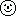on request
air motion transformer. The magnet system is based on Neodymium technology. The speaker has a 3 mm solid metal front plate.
• power handling (continuous/programme) = 120 W
• frequency range = 1800-41000 Hz
• resonance frequency fs = 2300 Hz
• impedance R = 8 Ohm
• sound pressure level SPL = 91 dB (2,83V; 1m)
• DC resistance Re = 8,1 Ohm
• effective piston radiating area Sd = 126 cm2
• overall diameter d = 104 mm
PDF data sheetMundorf AMT 1908C, D= 110 mm, schwarz       order no. M-amt1908cr110       EUR 209,00excl. VAT: € 175.63 / \$ 198.46
air motion transformer. The magnet system is based on Neodymium technology. The speaker has a 3 mm solid metal front plate.
• power handling (continuous/programme) = 120 W
• frequency range = 1800-41000 Hz
• resonance frequency fs = 2300 Hz
• impedance R = 8 Ohm
• sound pressure level SPL = 91 dB (2,83V; 1m)
• DC resistance Re = 8,1 Ohm
• effective piston radiating area Sd = 126 cm2
• overall diameter d = 110 mm
PDF data sheetMundorf AMT 1908C, D= 110 mm, silber       order no. M-amt1908crs110       EUR 209,00excl. VAT: € 175.63 / \$ 198.46
air motion transformer. The magnet system is based on Neodymium technology. The speaker has a 3 mm solid metal front plate.
• power handling (continuous/programme) = 120 W
• frequency range = 1800-41000 Hz
• resonance frequency fs = 2300 Hz
• impedance R = 8 Ohm
• sound pressure level SPL = 91 dB (2,83V; 1m)
• DC resistance Re = 8,1 Ohm
• effective piston radiating area Sd = 126 cm2
• overall diameter d = 110 mm
PDF data sheetopen a bigger photo

Mundorf AMT 21CM2.1-C       order no. M-amt21cm21-c       EUR 299,90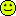excl. VAT: € 252.02 / \$ 284.78
air motion transformer. The magnet system is based on Neodymium technology. The speaker has a 3 mm solid metal front plate.
• power handling (continuous/programme) = 125 W
• frequency range = 1200-35000 Hz
• resonance frequency fs = 2300 Hz
• impedance R = 4 Ohm
• sound pressure level SPL = 93 dB (2,83V; 1m)
• DC resistance Re = 4,4 Ohm
• effective piston radiating area Sd = 126 cm2
• overall diameter d = 104 mm
PDF data sheetMundorf AMT 2109C, D= 94 mm, schwarz       order no. M-amt2109cr94       EUR 219,00excl. VAT: € 184.03 / \$ 207.96
air motion transformer. The magnet system is based on Neodymium technology. The speaker has a 3 mm solid metal front plate.
• power handling (continuous/programme) = 125 W
• frequency range = 1500-35000 Hz
• resonance frequency fs = 2100 Hz
• impedance R = 4 Ohm
• sound pressure level SPL = 93 dB (2,83V; 1m)
• DC resistance Re = 4,4 Ohm
• effective piston radiating area Sd = 126 cm2
• overall diameter d = 94 mm
PDF data sheetMundorf AMT 2109C, D= 94 mm, silber       order no. M-amt2109crs94       EUR 219,00excl. VAT: € 184.03 / \$ 207.96
air motion transformer. The magnet system is based on Neodymium technology. The speaker has a 3 mm solid metal front plate.
• power handling (continuous/programme) = 125 W
• frequency range = 1500-35000 Hz
• resonance frequency fs = 2100 Hz
• impedance R = 4 Ohm
• sound pressure level SPL = 93 dB (2,83V; 1m)
• DC resistance Re = 4,4 Ohm
• effective piston radiating area Sd = 126 cm2
• overall diameter d = 94 mm
PDF data sheet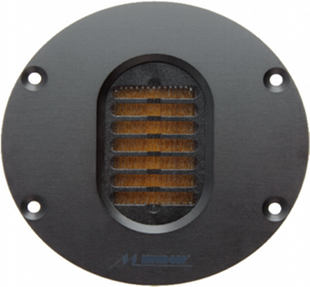open a bigger photo

Mundorf AMT 2109C, D= 104 mm, schwarz       order no. M-amt2109crs       EUR 219,00excl. VAT: € 184.03 / \$ 207.96
air motion transformer. The magnet system is based on Neodymium technology. The speaker has a 3 mm solid metal front plate.
• power handling (continuous/programme) = 125 W
• frequency range = 1500-35000 Hz
• resonance frequency fs = 2100 Hz
• impedance R = 4 Ohm
• sound pressure level SPL = 93 dB (2,83V; 1m)
• DC resistance Re = 4,4 Ohm
• effective piston radiating area Sd = 126 cm2
• overall diameter d = 104 mm
PDF data sheetMundorf AMT 2109C, D= 104 mm, silber       order no. M-amt2109c       EUR 219,00excl. VAT: € 184.03 / \$ 207.96
air motion transformer. The magnet system is based on Neodymium technology. The speaker has a 3 mm solid metal front plate.
• power handling (continuous/programme) = 125 W
• frequency range = 1500-35000 Hz
• resonance frequency fs = 2100 Hz
• impedance R = 4 Ohm
• sound pressure level SPL = 93 dB (2,83V; 1m)
• DC resistance Re = 4,4 Ohm
• effective piston radiating area Sd = 126 cm2
• overall diameter d = 104 mm
PDF data sheetMundorf AMT 2109C, D= 110 mm, schwarz       order no. M-amt2109cr110       EUR 219,00excl. VAT: € 184.03 / \$ 207.96
air motion transformer. The magnet system is based on Neodymium technology. The speaker has a 3 mm solid metal front plate.
• power handling (continuous/programme) = 125 W
• frequency range = 1500-35000 Hz
• resonance frequency fs = 2100 Hz
• impedance R = 4 Ohm
• sound pressure level SPL = 93 dB (2,83V; 1m)
• DC resistance Re = 4,4 Ohm
• effective piston radiating area Sd = 126 cm2
• overall diameter d = 110 mm
PDF data sheetMundorf AMT 2109C, D= 110 mm, silber       order no. M-amt2109crs110       EUR 219,00excl. VAT: € 184.03 / \$ 207.96
air motion transformer. The magnet system is based on Neodymium technology. The speaker has a 3 mm solid metal front plate.
• power handling (continuous/programme) = 125 W
• frequency range = 1500-35000 Hz
• resonance frequency fs = 2100 Hz
• impedance R = 4 Ohm
• sound pressure level SPL = 93 dB (2,83V; 1m)
• DC resistance Re = 4,4 Ohm
• effective piston radiating area Sd = 126 cm2
• overall diameter d = 110 mm
PDF data sheet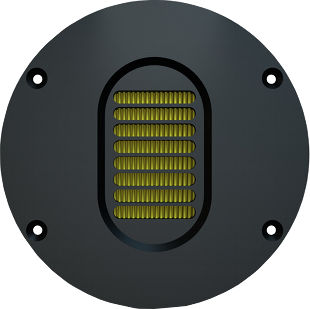open a bigger photo

Mundorf AMT 23CM1.1-C       order no. M-amt23cm11-c       EUR 369,90excl. VAT: € 310.84 / \$ 351.25
air motion transformer. The magnet system is based on Neodymium technology. The speaker has a 3 mm solid metal front plate.
• power handling (continuous/programme) = 125 W
• frequency range = 950-35000 Hz
• resonance frequency fs = 1700 Hz
• impedance R = 8 Ohm
• sound pressure level SPL = 90 dB (2,83V; 1m)
• DC resistance Re = 6,9 Ohm
• effective piston radiating area Sd = 175,5 cm2
• overall diameter d = 130 mm
PDF data sheetMundorf AMT 23CM1.1-R       order no. M-amt23cm11-r       EUR 369,90excl. VAT: € 310.84 / \$ 351.25
air motion transformer. The magnet system is based on Neodymium technology. The speaker has a 3 mm solid metal front plate.
• power handling (continuous/programme) = 125 W
• frequency range = 950-35000 Hz
• resonance frequency fs = 1700 Hz
• impedance R = 8 Ohm
• sound pressure level SPL = 90 dB (2,83V; 1m)
• DC resistance Re = 6,9 Ohm
• effective piston radiating area Sd = 175,5 cm2
• overall diameter d = 100x100 mm
PDF data sheetopen a bigger photo

Mundorf AMT 23CS1.1-C       order no. M-amt23cs11-c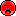- not available
air motion transformer. The magnet system is based on Neodymium technology. The speaker has a 3 mm solid metal front plate.
• power handling (continuous/programme) = 125 W
• frequency range = 900-32000 Hz
• resonance frequency fs = 1450 Hz
• impedance R = 8 Ohm
• sound pressure level SPL = 89 dB (2,83V; 1m)
• DC resistance Re = 7,3 Ohm
• effective piston radiating area Sd = 221 cm2
• overall diameter d = 130 mm
PDF data sheetMundorf AMT 23CS1.1-R       order no. M-amt23cs11-r- not available
air motion transformer. The magnet system is based on Neodymium technology. The speaker has a 3 mm solid metal front plate.
• power handling (continuous/programme) = 125 W
• frequency range = 900-32000 Hz
• resonance frequency fs = 1450 Hz
• impedance R = 8 Ohm
• sound pressure level SPL = 89 dB (2,83V; 1m)
• DC resistance Re = 7,3 Ohm
• effective piston radiating area Sd = 221 cm2
• overall diameter d = 100x100 mm
PDF data sheetopen a bigger photo

Mundorf AMT 2310C-C, D= 130 mm, schwarz       order no. M-amt2310cr       EUR 349,00excl. VAT: € 293.28 / \$ 331.40
air motion transformer. The magnet system is based on Neodymium technology. The speaker has a 3 mm solid metal front plate.
• power handling (continuous/programme) = 145 W
• frequency range = 900-32000 Hz
• resonance frequency fs = 1200 Hz
• impedance R = 8 Ohm
• sound pressure level SPL = 92 dB (2,83V; 1m)
• DC resistance Re = 8,4 Ohm
• effective piston radiating area Sd = 221 cm2
• overall diameter d = 130 mm
PDF data sheetMundorf AMT 2310C-C, D= 130 mm, silber       order no. M-amt2310crs       EUR 349,00excl. VAT: € 293.28 / \$ 331.40
air motion transformer. The magnet system is based on Neodymium technology. The speaker has a 3 mm solid metal front plate.
• power handling (continuous/programme) = 145 W
• frequency range = 900-32000 Hz
• resonance frequency fs = 1200 Hz
• impedance R = 8 Ohm
• sound pressure level SPL = 92 dB (2,83V; 1m)
• DC resistance Re = 8,4 Ohm
• effective piston radiating area Sd = 221 cm2
• overall diameter d = 130 mm
PDF data sheet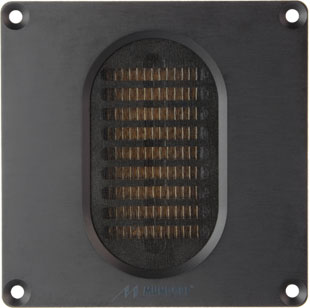open a bigger photo

Mundorf AMT 2310C-R, 100 mm x 100 mm, schwarz       order no. M-amt2310c       EUR 349,00excl. VAT: € 293.28 / \$ 331.40
air motion transformer. The magnet system is based on Neodymium technology. The speaker has a 3 mm solid metal front plate.
• power handling (continuous/programme) = 145 W
• frequency range = 900-32000 Hz
• resonance frequency fs = 1200 Hz
• impedance R = 8 Ohm
• sound pressure level SPL = 92 dB (2,83V; 1m)
• DC resistance Re = 8,4 Ohm
• effective piston radiating area Sd = 221 cm2
• overall diameter d = 100x100 mm
PDF data sheetMundorf AMT 2310C-R, 100 mm x 100 mm, silber       order no. M-amt2310cs       EUR 349,00excl. VAT: € 293.28 / \$ 331.40
air motion transformer. The magnet system is based on Neodymium technology. The speaker has a 3 mm solid metal front plate.
• power handling (continuous/programme) = 145 W
• frequency range = 900-32000 Hz
• resonance frequency fs = 1200 Hz
• impedance R = 8 Ohm
• sound pressure level SPL = 92 dB (2,83V; 1m)
• DC resistance Re = 8,4 Ohm
• effective piston radiating area Sd = 221 cm2
• overall diameter d = 100x100 mm
PDF data sheetopen a bigger photo

Mundorf AMT 25CM1.1-R       order no. M-amt25cm11-r       EUR 429,90excl. VAT: € 361.26 / \$ 408.22
air motion transformer. The magnet system is based on Neodymium technology. The speaker has a 3 mm solid metal front plate.
• power handling (continuous/programme) = 210 W
• frequency range = 900-35000 Hz
• resonance frequency fs = 1400 Hz
• impedance R = 8 Ohm
• sound pressure level SPL = 93 dB (2,83V; 1m)
• DC resistance Re = 7,3 Ohm
• effective piston radiating area Sd = 646,4 cm2
• overall diameter d = 100x130 mm
PDF data sheet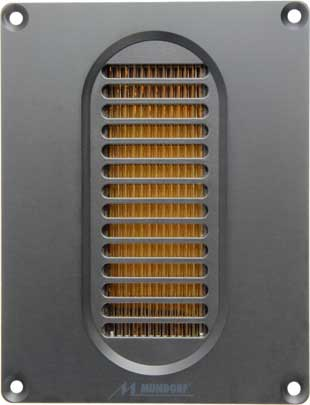open a bigger photo

Mundorf AMT 25CS2.1-R       order no. M-amt25cs21-r       EUR 429,90excl. VAT: € 361.26 / \$ 408.22
air motion transformer. The magnet system is based on Neodymium technology. The speaker has a 3 mm solid metal front plate.
• power handling (continuous/programme) = 230 W
• frequency range = 1000-32000 Hz
• resonance frequency fs = 1100 Hz
• impedance R = 8 Ohm
• sound pressure level SPL = 96 dB (2,83V; 1m)
• DC resistance Re = 7,6 Ohm
• effective piston radiating area Sd = 646,4 cm2
• overall diameter d = 100x130 mm
PDF data sheetMundorf AMT 25CM1.1-C       order no. M-amt25cm11-c       EUR 329,00excl. VAT: € 276.47 / \$ 312.41
air motion transformer. The magnet system is based on Neodymium technology. The speaker has a 3 mm solid metal front plate.
• power handling (continuous/programme) = 210 W
• frequency range = 900-35000 Hz
• resonance frequency fs = 1400 Hz
• impedance R = 8 Ohm
• sound pressure level SPL = 93 dB (2,83V; 1m)
• DC resistance Re = 7,3 Ohm
• effective piston radiating area Sd = 646,4 cm2
• overall diameter d = 150 mm
PDF data sheetopen a bigger photo

Mundorf AMT 25CS1.1-R       order no. M-amt25cs11-r       EUR 329,00excl. VAT: € 276.47 / \$ 312.41
air motion transformer. The magnet system is based on Neodymium technology. The speaker has a 3 mm solid metal front plate.
• power handling (continuous/programme) = 230 W
• frequency range = 800-31000 Hz
• resonance frequency fs = 1100 Hz
• impedance R = 8 Ohm
• sound pressure level SPL = 93 dB (2,83V; 1m)
• DC resistance Re = 7,8 Ohm
• effective piston radiating area Sd = 646,4 cm2
• overall diameter d = 100x130 mm
PDF data sheetMundorf AMT 25CS1.1-C       order no. M-amt25cs11-c       EUR 329,00excl. VAT: € 276.47 / \$ 312.41
air motion transformer. The magnet system is based on Neodymium technology. The speaker has a 3 mm solid metal front plate.
• power handling (continuous/programme) = 230 W
• frequency range = 800-31000 Hz
• resonance frequency fs = 1100 Hz
• impedance R = 8 Ohm
• sound pressure level SPL = 93 dB (2,83V; 1m)
• DC resistance Re = 7,8 Ohm
• effective piston radiating area Sd = 646,4 cm2
• overall diameter d = 150 mm
PDF data sheetMundorf AMT 25CS2.1-C       order no. M-amt25cs21-c       EUR 329,00excl. VAT: € 276.47 / \$ 312.41
air motion transformer. The magnet system is based on Neodymium technology. The speaker has a 3 mm solid metal front plate.
• power handling (continuous/programme) = 230 W
• frequency range = 1000-32000 Hz
• resonance frequency fs = 1100 Hz
• impedance R = 8 Ohm
• sound pressure level SPL = 96 dB (2,83V; 1m)
• DC resistance Re = 7,6 Ohm
• effective piston radiating area Sd = 646,4 cm2
• overall diameter d = 150 mm
PDF data sheet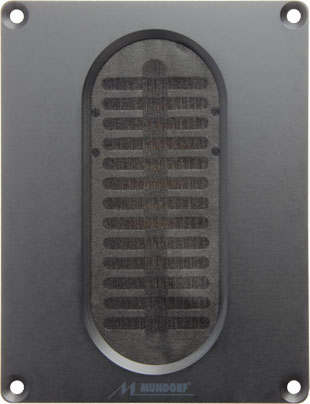open a bigger photo

Mundorf AMT 2510C-R, 100 mm x 130 mm, schwarz       order no. M-amt2510cr       EUR 429,00excl. VAT: € 360.50 / \$ 407.37
air motion transformer. The magnet system is based on Neodymium technology. The speaker has a 3 mm solid metal front plate.
• power handling (continuous/programme) = 230 W
• frequency range = 800-33000 Hz
• resonance frequency fs = 1100 Hz
• impedance R = 8 Ohm
• sound pressure level SPL = 97 dB (2,83V; 1m)
• DC resistance Re = 7,5 Ohm
• effective piston radiating area Sd = 646,4 cm2
• overall diameter d = 100x130 mm
PDF data sheetMundorf AMT 2510C-R, 100 mm x 130 mm, silber       order no. M-amt2510cs       EUR 429,00excl. VAT: € 360.50 / \$ 407.37
air motion transformer. The magnet system is based on Neodymium technology. The speaker has a 3 mm solid metal front plate.
• power handling (continuous/programme) = 230 W
• frequency range = 800-33000 Hz
• resonance frequency fs = 1100 Hz
• impedance R = 8 Ohm
• sound pressure level SPL = 97 dB (2,83V; 1m)
• DC resistance Re = 7,5 Ohm
• effective piston radiating area Sd = 646,4 cm2
• overall diameter d = 100x130 mm
PDF data sheetMundorf AMT 2510C-C, D= 150 mm, schwarz       order no. M-amt2510cr       EUR 429,00excl. VAT: € 360.50 / \$ 407.37
air motion transformer. The magnet system is based on Neodymium technology. The speaker has a 3 mm solid metal front plate.
• power handling (continuous/programme) = 230 W
• frequency range = 800-33000 Hz
• resonance frequency fs = 1100 Hz
• impedance R = 8 Ohm
• sound pressure level SPL = 97 dB (2,83V; 1m)
• DC resistance Re = 7,5 Ohm
• effective piston radiating area Sd = 646,4 cm2
• overall diameter d = 150 mm
PDF data sheetMundorf AMT 2510C-C, D= 150 mm,silber       order no. M-amt2510crs       EUR 429,00excl. VAT: € 360.50 / \$ 407.37
air motion transformer. The magnet system is based on Neodymium technology. The speaker has a 3 mm solid metal front plate.
• power handling (continuous/programme) = 230 W
• frequency range = 800-33000 Hz
• resonance frequency fs = 1100 Hz
• impedance R = 8 Ohm
• sound pressure level SPL = 97 dB (2,83V; 1m)
• DC resistance Re = 7,5 Ohm
• effective piston radiating area Sd = 646,4 cm2
• overall diameter d = 150 mm
PDF data sheet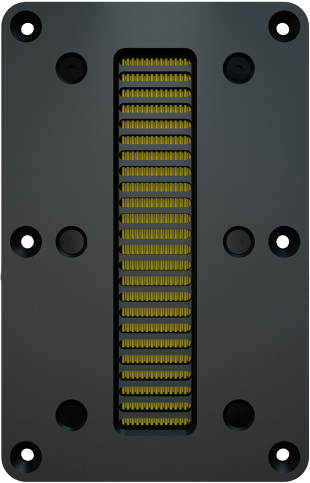open a bigger photo

Mundorf AMT 29CM1.1-R       order no. M-amt29cm11-r       EUR 699,90excl. VAT: € 588.15 / \$ 664.61
air motion transformer. The magnet system is based on Neodymium technology. The speaker has a 3 mm solid metal front plate.
• power handling (continuous/programme) = 250 W
• frequency range = 1000-32000 Hz
• resonance frequency fs = 1500 Hz
• impedance R = 8 Ohm
• sound pressure level SPL = 100 dB (2,83V; 1m)
• DC resistance Re = 8,5 Ohm
• effective piston radiating area Sd = 651,5 cm2
• overall diameter d = 125x195 mm
PDF data sheetMundorf AMT 29CM2.1       order no. M-amt29cm21       EUR 429,00excl. VAT: € 360.50 / \$ 407.37
air motion transformer.open a bigger photo

Mundorf AMT 164UM2.1-R       order no. M-amt164um21-ron request
air motion transformer. The magnet system is based on Neodymium technology. The speaker has a 3 mm solid metal front plate.
• power handling (continuous/programme) = 250 W
• frequency range = 1000-35000 Hz
• resonance frequency fs = 1500 Hz
• impedance R = 8 Ohm
• sound pressure level SPL = 98 dB (2,83V; 1m)
• DC resistance Re = 8,7 Ohm
• effective piston radiating area Sd = 651,5 cm2
• overall diameter d = 90x182 mm
PDF data sheetopen a bigger photo

Mundorf AMT 164UM1.1-R       order no. M-amt164um11-r       EUR 549,00excl. VAT: € 461.34 / \$ 521.32
air motion transformer. The magnet system is based on Neodymium technology. The speaker has a 3 mm solid metal front plate.
• power handling (continuous/programme) = 240 W
• frequency range = 1200-36000 Hz
• resonance frequency fs = 2000 Hz
• impedance R = 8 Ohm
• sound pressure level SPL = 97 dB (2,83V; 1m)
• DC resistance Re = 8,4 Ohm
• effective piston radiating area Sd = 651,5 cm2
• overall diameter d = 90x182 mm
PDF data sheet

## Dipol AMTopen a bigger photo

Mundorf AMT 17D1.1       order no. M-amt17d11       EUR 149,00excl. VAT: € 125.21 / \$ 141.49
air motion transformer. The magnet system is based on Neodymium technology.
• power handling (continuous/programme) = 60 W
• frequency range = 1200-29000 Hz
• resonance frequency fs = 2100 Hz
• impedance R = 8 Ohm
• sound pressure level SPL = 89 dB (2,83V; 1m)
• DC resistance Re = 8,6 Ohm
• effective piston radiating area Sd = 126 cm2
• overall diameter d = 55x60 mm
PDF data sheetopen a bigger photo

Mundorf AMT 17D2.1       order no. M-amt17d21- not available
air motion transformer. The magnet system is based on Neodymium technology.
• power handling (continuous/programme) = 60 W
• frequency range = 1500-35000 Hz
• resonance frequency fs = 2000 Hz
• impedance R = 4 Ohm
• sound pressure level SPL = 91 dB (2,83V; 1m)
• DC resistance Re = 3,9 Ohm
• effective piston radiating area Sd = 126 cm2
• overall diameter d = 55x60 mm
PDF data sheet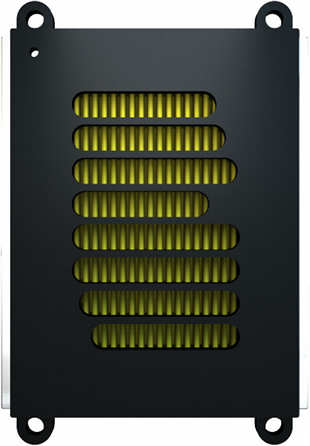open a bigger photo

Mundorf AMT 23D1.1       order no. M-amt23d11       EUR 279,00excl. VAT: € 234.45 / \$ 264.93
air motion transformer. The magnet system is based on Neodymium technology.
• power handling (continuous/programme) = 92 W
• frequency range = 1200-30000 Hz
• resonance frequency fs = 1400 Hz
• impedance R = 8 Ohm
• sound pressure level SPL = 89 dB (2,83V; 1m)
• DC resistance Re = 6,9 Ohm
• effective piston radiating area Sd = 221 cm2
• overall diameter d = 61x98 mm
PDF data sheet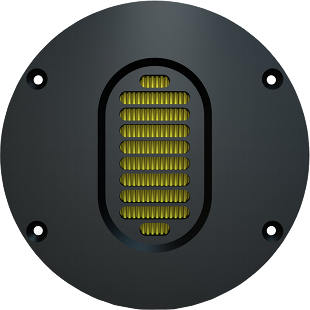open a bigger photo

Mundorf AMT 23D6.1-C       order no. M-amt23d61-c       EUR 369,90excl. VAT: € 310.84 / \$ 351.25
air motion transformer. The magnet system is based on Neodymium technology. The speaker has a 3 mm solid metal front plate.
• power handling (continuous/programme) = 92 W
• frequency range = 1200-30000 Hz
• resonance frequency fs = 1500 Hz
• impedance R = 8 Ohm
• sound pressure level SPL = 88 dB (2,83V; 1m)
• DC resistance Re = 6,9 Ohm
• effective piston radiating area Sd = 221 cm2
• overall diameter d = 130 mm
PDF data sheetMundorf AMT 23D6.1-R       order no. M-amt23d61-r       EUR 369,90excl. VAT: € 310.84 / \$ 351.25
air motion transformer. The magnet system is based on Neodymium technology. The speaker has a 3 mm solid metal front plate.
• power handling (continuous/programme) = 92 W
• frequency range = 1200-30000 Hz
• resonance frequency fs = 1500 Hz
• impedance R = 8 Ohm
• sound pressure level SPL = 88 dB (2,83V; 1m)
• DC resistance Re = 6,9 Ohm
• effective piston radiating area Sd = 221 cm2
• overall diameter d = 100x100 mm
PDF data sheet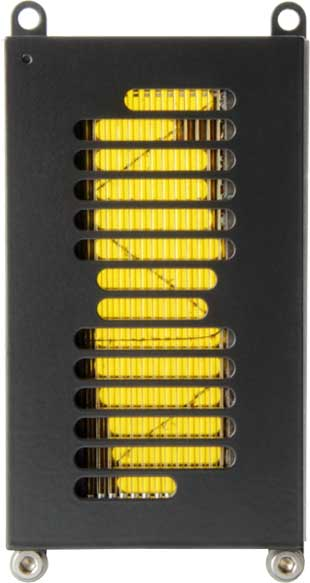open a bigger photo

Mundorf AMT 25D1.1       order no. M-amt25d11       EUR 429,90excl. VAT: € 361.26 / \$ 408.22
air motion transformer. The magnet system is based on Neodymium technology.
• power handling (continuous/programme) = 135 W
• frequency range = 1100-30000 Hz
• resonance frequency fs = 1250 Hz
• impedance R = 6 Ohm
• sound pressure level SPL = 92 dB (2,83V; 1m)
• DC resistance Re = 5,6 Ohm
• effective piston radiating area Sd = 646,4 cm2
• overall diameter d = 65x128 mm
PDF data sheetopen a bigger photo

Mundorf AMT 25D6.1-R       order no. M-amt25d61-r       EUR 429,90excl. VAT: € 361.26 / \$ 408.22
air motion transformer. The magnet system is based on Neodymium technology. The speaker has a 3 mm solid metal front plate.
• power handling (continuous/programme) = 135 W
• frequency range = 1100-30000 Hz
• resonance frequency fs = 1400 Hz
• impedance R = 6 Ohm
• sound pressure level SPL = 93 dB (2,83V; 1m)
• DC resistance Re = 5,6 Ohm
• effective piston radiating area Sd = 646,4 cm2
• overall diameter d = 100x130 mm
PDF data sheetMundorf AMT 25D6.1-C       order no. M-amt25d61-c       EUR 429,90excl. VAT: € 361.26 / \$ 408.22
air motion transformer. The magnet system is based on Neodymium technology. The speaker has a 3 mm solid metal front plate.
• power handling (continuous/programme) = 135 W
• frequency range = 1100-30000 Hz
• resonance frequency fs = 1400 Hz
• impedance R = 6 Ohm
• sound pressure level SPL = 93 dB (2,83V; 1m)
• DC resistance Re = 5,6 Ohm
• effective piston radiating area Sd = 646,4 cm2
• overall diameter d = 150 mm
PDF data sheet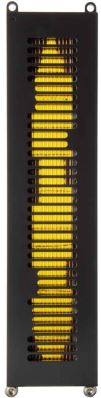open a bigger photo

Mundorf AMT 27D1.1       order no. M-amt27d11       EUR 699,90excl. VAT: € 588.15 / \$ 664.61
air motion transformer. The magnet system is based on Neodymium technology.
• power handling (continuous/programme) = 190 W
• frequency range = 1000-29000 Hz
• resonance frequency fs = 1150 Hz
• impedance R = 12 Ohm
• sound pressure level SPL = 97 dB (2,83V; 1m)
• DC resistance Re = 13,5 Ohm
• effective piston radiating area Sd = 891 cm2
• overall diameter d = 59x258 mm
PDF data sheet
The cabinet volume calculations are executed considering an external resistance of 0.3 Ohm. We are using, if no other data is known or available, the information supplied by the manufacturer.

Please note: the frequency response doesn't say much about the cut-off frequency inside the cabinet! Therefore, we mostly give you 2 examples with dimensioning considerations and an indication, how low the loudspeaker will reproduce.

Home